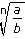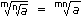Exam Mission

Exam Information| Govt Jobs.| Exam Preparation | Exam Study Material |Exam Date|Material PDF

1. Laws of Indices:
1. am x an = am + n
2.  am = am - n an
3. (am)n = amn
4. (ab)n = anbn
5.an = an b bn
6. a0 = 1
2. Surds:
Let a be rational number and n be a positive integer such that a(1/n) = a
Then, a is called a surd of order n.
3. Laws of Surds:
1. a = a(1/n)
2. ab = a x b
3.= a b
4. (a)n = a
5.6. (a)m = am